American Journal of Aerospace Engineering
Volume 3, Issue 2, April 2016, Pages: 6-12

On Optimal Trajectory in Space Flight

Sergey Orlov

Petrozavodsk State University, Petrozavodsk, Russia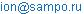Sergey Orlov. On Optimal Trajectory in Space Flight. American Journal of Aerospace Engineering. Vol. 3, No. 2, 2016, pp. 6-12. doi: 10.11648/j.ajae.20160302.11

Received: July 18, 2016; Accepted: March 21, 2016; Published: April 12, 2016

Abstract: Are investigated a trajectory of new type in distant, space flights unlike usual trajectories of direct flight to heavenly object (Moon) it is supposed to use asymmetry of a gravitational field and to carry out flight bypassing the most power gravitational impact on the spacecraft. It leads to economy of power for 20-30%.

Keywords: The Theory of Vortex Gravitation, Space Flights with an Optimum Trajectory

1. Introduction

Now in a science there is a statement that force of gravitation is created by any body. According to this law force of gravitation decreases under the law of a return square of distance from this body equally in all directions.

In the theory of vortex gravitation  proposed study, the force of gravity - a force push, which is caused by the decrease in pressure in the space medium called ether. In turn pressure reduction in heavenly points is caused by vortex rotation of ether round these points, according to hydro aerodynamics laws. Speed of orbital rotation of streams of each whirlwind is inversely proportional to distance from square of center of this whirlwind.

As the whirlwind rotates in one plane, and the law of dependence of speed, pressure and force of gravitation from square of distance to the center of rotation of ether, operates too only in one plane of rotation of ether.

2. Model of the Origin of the Universal Gravitation Force

In this section, a model of appearance of the gravitation attraction force is considered from the viewpoint of aerodynamics. Namely, the two-dimensional model (Fig. 1) is considered on the basis of the following initial postulates. These postulates will be expanded and defined more exactly below.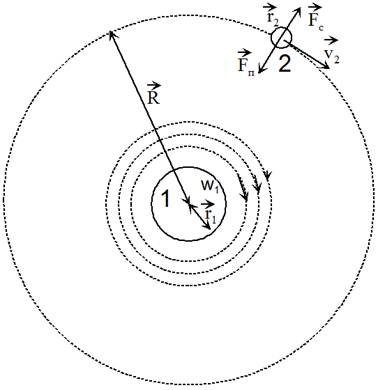Fig. 1. Two-dimensional model of gravitational interaction of two bodies. The forces are shown acting on body 2: Fc – the centrifugal force, Fп – the force of attraction of body 2 from body 1; v2 – linear velocity of body 2 at the orbit, R – the radius of the orbit, r1 – the radius of body 1, r2 – the radius of body 2, w1 – angular velocity of ether rotation at the surface of body 1, and m2 are the mass of body 2.

1. There exists an ether vortex around any physical object.

2. The ether motion in the vortex has laminar nature and obeys the laws of hydro- or aero-dynamics; the ether viscosity is low.

3. The pressure gradient, arising during the vortex motion of the ether gas, is the reason for an attractive force from body 1 to body 2 (see Fig. 1).

4. The direction of the force Fп does not depend on the direction of the ether angular velocity, which is necessary for the attractive force between the bodies, irrespective of their relative position. This implies the absence of the Magnus force – the force of interaction between the two vortexes which appears in the classical aerodynamics. Such an assumption can take place at a weak interaction between the two ether flows, as if they would move one through another, not affecting mutual motion.

5. The appearing attraction force must describe the experimentally obtained law of gravity: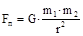(1)

where m1, m2 are the masses of bodies 1 and 2, respectively, G=6.672 ∙10-11 N∙m2/kg2 – the gravitation constant, and r – the distance between the bodies.

Next we consider the appearance of the attraction force in more detail and derive a formula describing it. As was said above, a pressure gradient arises as the result of the vortex motion. Let’s find the radial distribution of the pressure and the ether velocity. For this purpose, we write the Navier-Stokes equation for the motion of a viscous liquid (gas).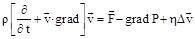(2)

where r is the ether density,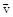and P are, respectively, its velocity and pressure, and h - the ether viscosity. In cylindrical coordinates, taking into account the radial symmetry vr=vz=0, vj=v(r), P=P(r), the equation can be written as the system:(3)

In case of a compressible substance (ether), there will be a function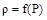(instead of r).

From the first equation of system (3), one can find P(r) provided that the dependence v(r) is known. The latter, in turn, should be found from the second equation of that same system (one of the solution of which is the function v(r) ~ 1/r). At zero viscosity, the system permits any dependence v(r) .

The force affecting the body can be estimated from the formula(4)

where V is the volume of body 2.

In cylindrical coordinates the modulus of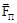is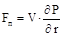(5)

Then, comparing equations (3) and (5), for the incompressible ether (r=const) we find that(6)

For the correspondence of the ether rotation to the planet motion law (according to Kepler 3-rd law) in one cosmic (e.g., Solar) system, v(r) must obey the dependence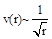, and not the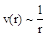.

Taking into account the edge condition v (r1) = w1∙r1,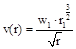(7)

Thus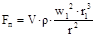(8)

Here we make one more supposition (№ 6) – Ether penetrates through all the space, including the physical bodies. The volume V in formula (8) is an effective volume, i.e. the volume of elementary particles, which the body is composed of. All the bodies are composed of electrons, protons, and neutrons. The radius of an electron is much smaller than that of a proton and neutron. The radii of the latter are approximately equal to each other, rn ~ 1.2∙10-15 m. The same is true as to the masses: mn~1.67∙10-27 kg (rn and mn are the radius and the mass of a nucleon). Therefore, the volume in formula (8) is: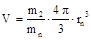(9)

Taking into account the formula (9), Eq. (8) can be rewritten as(10)

From the obtained formula for vortex gravitation, it is obvious that, in the existing Newton’s law of gravitation, instead of the reason of gravity (the gradient of pressure), the consequence of that (i.e. the mass) is used.

3. Cause of the Ellipsoidal Shape of Orbits

It is known that the planets circulate around the Sun by an ellipse with a small eccentricity.

This fact is accounted for from the viewpoint of vortex gravitation; moreover, it serves as a convincing proof of the existence of this gravitation with its discoid plane-symmetrical configuration (Section 2).

The cause of the planets orbit "compression" is the inclination of these orbits to the sun torsion plane. This statement is based on the following.

As is known, the planes of orbital motion of all the planets are situated with small deviations one from another. Consequently, planet orbit planes have inclinations to the plane of the sun gravitation torsion, where the highest gravitation force for this orbit acts, and the planets should intersect the sun torsion in two points during their orbital motion. As will be shown below, these intersection points coincide with the centers of perihelion and aphelion.

In the aphelion and perihelion, the sun gravitation force acts onto the planets with the highest magnitude at this orbit, and hence the orbit possesses a maximum curvature. At going out (deviation) from the sun torsion plane, the gravitation forces decrease and the planet trajectory "unbends" (Fig. 2). As such the cycle of the gravitation force and motion trajectory change repeats for each planet and for each turn around the Sun. The more the planet circulation trajectory is deviated from the central sun torsion plane, the higher is the degree of the gravitation force decrease in these regions, and hence the higher is the degree of "straightening" or "compression" of the orbit. Due to a permanent cyclic change of these forces, the orbit becomes ellipsoidal.

At significant inclinations and high speeds, the orbit of a satellite (meteorite, comet) have a hyperbola or parabola trajectory, and, correspondingly, the celestial object, once turning around the Sun, abandons the sun gravitation torsion field forever.

Determining of the sun torsion direction

On the basis of the stated above, it is obvious that the orbit trajectory eccentricity value of any planet depends on the value of inclination of this orbit to the sun torsion. Therefore, a reverse relation takes place, i.e. the lower the orbit eccentricity, the lower the inclination of the planet orbital plane to the sun torsion plane.

Since the Venus orbit has the least eccentricity, for preliminary calculations, it is permissibly to accept the following property of the sun torsion:

- the direction of the sun gravitation torsion in the World coordinates coincides with the Venus orbital plane direction to a highest degree.

Therefore, all the inclinations and latitudes of any astronomical point can be determined with regard to the orbital plane of Venus with a small correction up to 0.5 degree.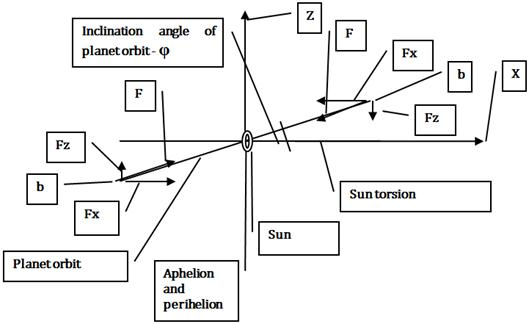Fig. 2. Kinematical scheme of orbital motion.

Let’s consider the planet circulation in more detail with the Mercury motion as an example, in accordance with its heliocentric coordinates of 1993 .Fig. 3. Orbit of Mercury.

In Table 1 and Fig’s 2 and 3, the following denotations are used:

Z – the torsion rotation axis

№ - numbers of the Mercury orbit points according to the astronomy calendar of 1993

Д – the heliocentric longitude J2000.0 of these points

r – the radius-vector, in A. U.

d – the distance from the ellipse center to the point under study, in million km.

V – the orbital speed, in km/s

R – the curvature radius, in million km, R = a2b2/ d3where а, в are the major and minor axes

Fc – the centrifugal forces

Fg – the gravitation forces.

The values of centrifugal and gravitation forces are in portions of the planet mass.

О – О – the apse line coinciding with the line of intersection of the Mercury orbital plane with sun torsion plane. The center of Mercury perihelion has the longitude of (85.83 – 8.18) degree regarding to the point №1 in 1993.

On the basis of comparison of latitudes from the astronomy calendar, it has been established that the Mercury traverses the Sun (Venus) torsion in the aphelion and perihelion. The same is true for the other planets. Therefore, at these parts of the planet motion, the orbit curvatures are maximum and equal to each other, and the gravitation forces correspond to their classical values, i.e. they are inversely proportional to square of the distance to the Sun or are equal to the centrifugal forces.

Thus, the exact position of the sun torsion by its latitude relatively to the ecliptic is indicated by the latitudes of the aphelion and perihelion centers of each planet and by the apse line directions of these planets.

Comparing the astronomy point latitude values with the ratio of the gravitation and centrifugal forces in these space points, one can find that the more the planet orbit is inclined to the sun torsion, the higher is the difference between the Newtonian calculated gravitation forces and the actual centrifugal forces in those same points.

We consider two points of the Mercury orbit (№ 9 и № 10 in Fig. 3).

Table 1. Mercury orbit parameters.

 № Д, degree r d V R Fc Fg 9 250,04 0,4657 58,11 38,41 55,46 26,60 27,32 10 263,78 0,4659 58,03 38,96 55,69 27.26 27,29

The distance between point 10 and the Sun is 0.4659 х 150 = 69.885 million km.

For point 9 it is - 0.4657 х 150 = 69.855 million km.

The distance of point 10 from the Sun is 1.0004 times longer than that for point 9. Therefore, in point 10, the Newtonian sun gravitation forces have to be 1.001 times less than those in point 9 (see Table 1). In reality, according to the calculation, the value of centrifugal forces in point 10 are 1.025 times higher as compared to point 9 which is associated with a larger orbit curvature in this point (see Table 1). Since the planet circulation centrifugal forces are reactive and always equal to the gravitation forces, it is follows from the above-said that, in this region of Mercury motion trajectory, the classical gravitation law is not fulfilled.

On the basis of the vortex gravitation model with a plane-symmetrical configuration, this paradox has a physical-mathematical ground.

The Newton’s world attraction law or formula 10 in Section 2 can describe the action of the gravitation forces only in the plane of the gravitation torsion.

The above-presented calculation of the centrifugal forces appearing at the planet motion in the aphelion shows that the inertial circulation of the planets along an ellipsoidal trajectory in a central-symmetrical gravitation field is impossible in accordance with the classical ideas.

It should be noted that the planet orbit perihelion revolving round the Sun is also accounted for by a permanent change of the force magnitudes acting upon the planets.

4. Calculation of Gravitation in Three-Dimensional Model

The change of the dynamical properties of the planets at their inclination, discussed in Section 3, gives a possibility to obtain a formula describing the change of gravitation forces in the three-dimensional model.

Comparing the orbit compression coefficients for all the planets with cosine of the angle of inclination of these orbits to the sun torsion, one finds that these values are directly proportional to each other:

b/a ~ Cos φ                                (11)

Proofs of equation (11)

axis Х – the direction of the parent torsion central plane.

axis Z – the rotation axis of the parent torsion.

φ – the inclination angle of the satellite (planet) orbit torsion.

ОВ – the curvature radius of the torsion-satellite revolving at the coincidence of the satellite-torsion motion trajectory with the parent torsion rotation plane, i.e. at the perihelion or aphelion, or at the apex of the orbit major semiaxis:

ОВ = b2 / а                                (12)

OD1 - the curvature radius of the torsion-satellite revolving when it moves in a region possessing the inclination of angle φ from the parent torsion central plane, i.e. at the apex of the orbit minor semiaxis:

OD1 = а2 / b                               (13)

We prove that the equation cos φ = b/а is fulfilled at equalities (12) and (13)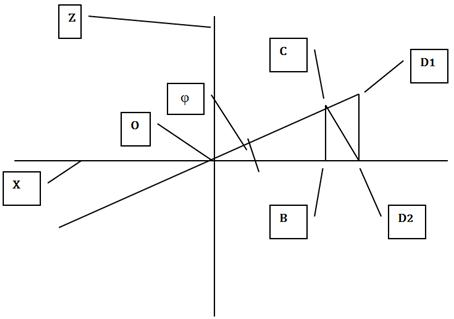Fig. 4. Plane projections of minor and major orbital semiaxes.

Proof:

First we draw a segment ОВ on the axis Х (fig. 4) coinciding with the apses line. This segment is to be equal to the curvature radius in the major semiaxis apex and is directed along the sun torsion central plane or the apses line.

Let’s now draw a line from the center О with the angle φ; the direction of this line has to coincide with the minor semiaxis apex.

Since, from the problem condition, cos φ = b/a = OB/OC, then:

OC = OB а/b = (b2/а) (a/b) = b

Let’s drop a perpendicular from point C on axis X, as the angle OCD2 is right:

OC/OD2 = cos φ = b/а, whence

OD2 = OC а/b = b (a/b) = a,

And finally we drop a perpendicular from point D2 on line ОС, as the angle D1D2O is right:

OD2 / OD1 = cos φ = b/a, whence OD1 = OD2 (a/b) = (a2/b)

Therefore, equations (12) and (13) are fulfilled provided that cos φ = b/а. That is, the cosine of the planet orbit inclination angle in the minor semiaxis apex to the sun torsion plane is equal to the compression coefficient of this orbit.

Note 1. The inclination φ of an orbital point does not coincide with the angle of inclination of this point indicated in astronomy calendars, because, according to the astronomy rules, all the coordinates in the Solar system are measured heliocentrically and from the ecliptic plane.

Since the centrifugal forces are reactive and always equal to the sun attraction forces, these centrifugal forces may be considered as experimental or etalon values for the estimation of the accuracy and correctness of the results of gravitation forces calculations. Therefore, the change of the value of the planet centrifugal forces at a change of their coordinates is always equal to the change of the value of the gravitation force acting onto this planet.

Determining of the three-dimensional gravitation coefficient Kg.

Let’s write the formulas to determine the orbit (ellipse) curvature radius:

- in the major semiaxis apex or in perihelion and aphelion:

Rкр.а = b2 / а                              (14)

- in the minor semiaxis apex:

Rкр.в = а2 / b                              (15)

On the basis of the 2nd Kepler law, the planets change the orbital velocity (V) as a function of the distance to the Sun (R), in the limits of their orbits, in the following proportion:

Va ~ 1/ Ra Vb ~ 1/ Rb                      (16)

where

Va – the orbital speed in the perihelion (aphelion), i.e. in the apex of the planet orbit major semiaxis,

Vb – the orbital speed in the apex of the planet orbit minor semiaxis

Ra – the distance from the Sun to the aphelion (perihelion).

Rb – the distance from the Sun to minor semiaxis apex.

The centrifugal force is determined from the formula:

Fc = m V2 / Rкр                         (17)

Substituting (14) – (16) into (17):

Fca = m Va2 / Rкр.а ~ m a /Ra2 b2             (18)

Fcb = m Vb2 / Rкр.b ~ m b / Rb2 a2            (19)

Since the gravitation forces in the aphelion and perihelion Fa correspond to their classical values or to the centrifugal forces, then, to determine a deviation of the gravitation forces in the torsion periphery (in the minor semiaxis apex – point b), it is necessary to determine the analogous deviation of the values of the centrifugal forces as compared to those same forces in the perihelion. For this purpose, we divide formula (19) by formula (18):

Fcb / Fca = [b3 / a3] [Ra2 / Rb2]

Here the relative value Ra2 / Rb2, in accordance with formula 10 in Section 2 or with the Newton formula, determines the gravitation force change as a function of the change of the distance from the torsion center to the points under consideration.

According to the expression (11), the value b/a equals to the cosine of the inclination angle in the considered point. Hence, this value determines the change of the gravitation forces as a function of the inclination of the considered point to the sun torsion.

Therefore, one can write:

b3/a3 = Cos3 φ = Kg                           (20)

The gravitation forces in any point of the cosmic space are determined by the formula:

Fv = Fg Cos3 φ,                            (21)

where

Fg – the gravitation force in the two-dimensional model (formula 10 in Section 2 or Newton equation)

Fv – the gravitation force in the three-dimensional model

Consequently, using the gravitation coefficient Kg, one can determine the gravitation forces in any point distant from the center of a cosmic torsion.

Formula (21) shows that, when moving away from the gravitation torsion plane, parallel to the torsion axis, the gravitation force decrease inversely as the cube of the distance - 1/s3

5. Calculation of Vortex Gravitation

In general, the force of gravity can be calculated by the formula 21 -

Fgv = Fgn Cos3 φ

where

Fgn - the force of gravity in the two-dimensional model (Eq. 10 in , which corresponds to the empirical formula for the law of universal gravitation Newton)

Fgv - the force of gravity in a three-dimensional vortex model.

φ - the angle between the straight line connecting the center of the torsion from this point, and the plane gravitational torsion.

The location of the plane of cosmic torsion can determine the coordinates of celestial bodies - satellites of the torsion.

In the solar system, the heliocentric latitude, the gravitational torsion coincide with latitude of the center of the perihelion and aphelion of the orbits of all the planets.

The earth latitude, the gravitational torsion coincide with latitudes of apogee and perigee of the orbit of the moon.

Thus, the coordinates of the gravitational torsion, we can determine the coordinates of the plane in which the gravitational force decreases at the lower, that is inversely proportional to the square of the distance from the center of torsion. As Earth is in the center earht gravitational torsion, at removal from it at distant space flights it is necessary to move to detour earth torsion, instead of on a direct trajectory, as in case of flight on the Moon.

The following shows the calculation of the physical work required to make the spacecraft during flight to the moon in two different routes.

Let’s consider a problem of comparing the works expended on getting over the gravitation attraction forces (F) by a body, when traveling from point A to point C (see Fig. 5) by the paths AC and ABC at two different F(r, j) dependences. The OAS line – a face projection gravitational torsion of Earth.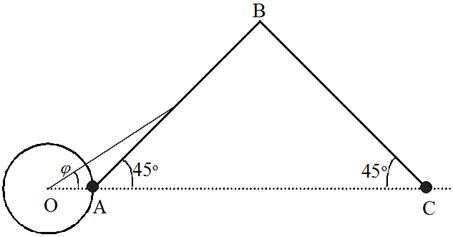Fig. 5. Scheme of space flight.

O – centre of Earth

A – start of flight

C – Moon (finish)

AC – projection of gravitational flat

In the first case, F is independent of j and obeys the Newton law(22)

where m1 and m2 are the masses of bodies, G – the gravitation constant, and r – the distance between the bodies.

In the second case, F depends on j in accordance with formula (21)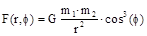(23)

where j is the angle between axis ОС and the position radius-vector of the replaced body.

As is known, the work equals to the path integral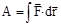(24)

Let AAC be the work expended at the transference AC for the case of the dependence (22). We determine the works AAB and A. For A’AC being the work expended at the transference AC for the case of the dependence (23) we determine, respectively, the works A’AB and A’.

Now we write the integral (24) for each case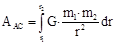(25)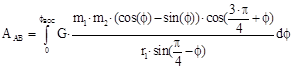(26)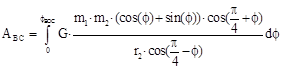(27)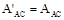(28)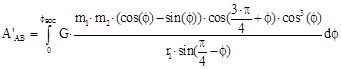(29)(30)

where r1 – the distance ОА, r2 – ОС, and jBOC – the angle BOC.

Formula (28) is valid because, in this direction, the forces (22) and (23) are equal to each other.

Calculating the integrals (28-30) numerically for the case of moonflight (r1=6400∙103 m, r2= 40000000m, m2=6∙1024 kg, m1=1 kg), one obtains AAC = 6.1554643∙107 J, AAB = 6.1140242∙107 J, ABC = 4.1440045∙104 J, A’AB = 4.5279719 ∙107 J, A’BC = 3.5727542∙105 J.

One can see that AAC= AAB +ABC, which just must be the case for the Newtonian forces when the work does not depend on the transference path from point A to point С.

In the case of the law (23), the work on the path ABC equals to A’ABС= A’AB +A’BC= 4.5636994∙107 J. This is less than the work A’ = AAC = 6.1554643∙107 J.

The ratio (decrease) of the works is s=A’ABС / AAC = 0.7414062. The value of s depends on the distances r1 and r2 and on the transference path.

Thus, the transference by the path ABC in the case of the law (23) is more energetically preferable than that directly by the path AC.

6. Conclusion

The above calculation shows that the moonflight with a detour of the Earth torsion should decrease the fuel consumption on 25%.

At present, most interplanetary cosmic apparatus get accelerations which can not be explained on the basis of cosmic calculations in the relativity theory of Einstein. Particularly, deviations have been found for the apparatus of «Galileo», «Rosetta» and «Cassini». The suggested model of vortex gravitation (formula 21) shows that, if the trajectory of the satellite flight does not coincide with the Sun gravitation torsion plane, then one should take into account the value of gravitation coefficient in the calculation of solar gravity acting onto the satellites. This coefficient (Cos3j) reduces the value of solar gravity, which gives a certain acceleration to cosmic satellites and results in a deviation of the motion trajectory.

References

1. S. Orlov. Foundation of vortex gravitation, cosmology and cosmogony. Global journal of science Frontier research. Physic and Space Science Volume 12 issue 1 Version 1.0 January 2012https://globaljournals.org/GJSFR_Volume12/3-Foundation-of-vortex-gravitation-cosmology.pdf
2. L V Kiknadze, Yu G Mamaladze. Classical hydrodynamics for physicists-experimentalists. Tbilisi University Press. Tbilisi, Georgia. 1979. Page 136.
3. A P Gulyaev. Astronomy calendar. Cosmosinform. Moscow, Russia. 1993. Page 285.

 Contents 1. 2. 3. 4. 5. 6.
Article ToolsAbstractPDF(750K)